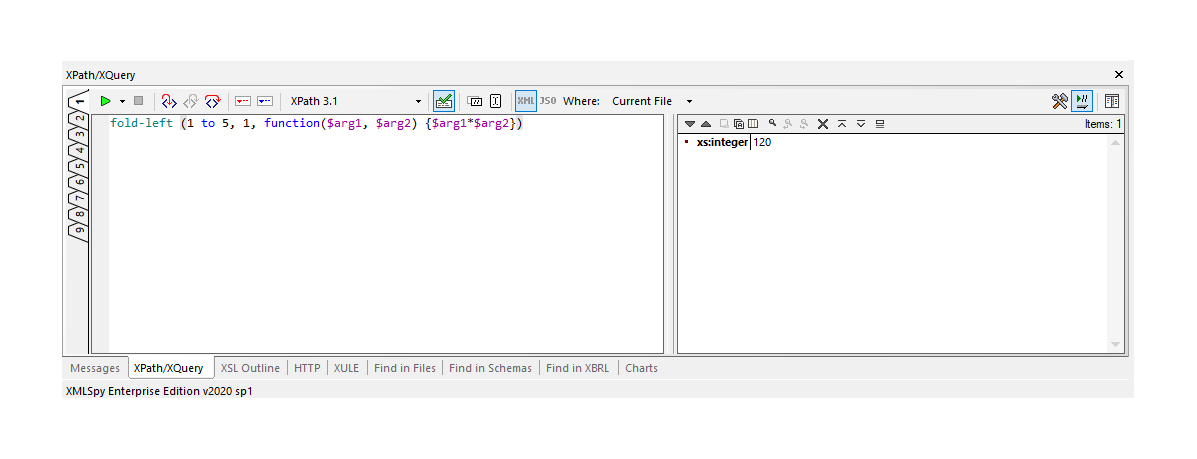## XPath/XQuery fold-left function

### Summary

Processes the supplied sequence from left to right, applying the supplied function repeatedly to each item in turn, together with an accumulated result value.

### Signature

`fn:fold-left`(
`\$seq` as `item()*`,
`\$zero` as `item()*`,
`\$f` as `function(item()*, item()) as item()*`
) as `item()*`

### Properties

This function is deterministic, context-independent, focus-independent, and higher-order.

### Rules

The effect of the function is equivalent to the following implementation in XQuery:

```declare function fn:fold-left(
\$seq as item()*,
\$zero as item()*,
\$f as function(item()*, item()) as item()*)
as item()* {
if (fn:empty(\$seq))
then \$zero
};```

or its equivalent in XSLT:

```<xsl:function name="fn:fold-left" as="item()*">
<xsl:param name="seq" as="item()*"/>
<xsl:param name="zero" as="item()*"/>
<xsl:param name="f" as="function(item()*, item()) as item()*"/>
<xsl:choose>
<xsl:when test="fn:empty(\$seq)">
<xsl:sequence select="\$zero"/>
</xsl:when>
<xsl:otherwise>
</xsl:otherwise>
</xsl:choose>
</xsl:function>```

### Examples

The expression ```fn:fold-left(1 to 5, 0, function(\$a, \$b) { \$a + \$b })``` returns `15`.

The expression ```fn:fold-left((2,3,5,7), 1, function(\$a, \$b) { \$a * \$b })``` returns `210`.

The expression ```fn:fold-left((true(), false(), false()), false(), function(\$a, \$b) { \$a or \$b })``` returns `true()`.

The expression ```fn:fold-left((true(), false(), false()), false(), function(\$a, \$b) { \$a and \$b })``` returns `false()`.

The expression ```fn:fold-left(1 to 5, (), function(\$a, \$b) {(\$b, \$a)})``` returns `(5,4,3,2,1)`.

The expression `fn:fold-left(1 to 5, "", fn:concat(?, ".", ?))` returns `".1.2.3.4.5"`.

The expression `fn:fold-left(1 to 5, "\$zero", fn:concat("\$f(", ?, ", ", ?, ")"))` returns `"\$f(\$f(\$f(\$f(\$f(\$zero, 1), 2), 3), 4), 5)"`.

The expression `fn:fold-left(1 to 5, map{}, function(\$map, \$n) {map:put(\$map, \$n, \$n*2)})` returns `map{1:2, 2:4, 3:6, 4:8, 5:10}`.### Error Conditions

As a consequence of the function signature and the function calling rules, a type error occurs if the supplied function \$f cannot be applied to two arguments, where the first argument is either the value of \$zero or the result of a previous application of \$f, and the second is any single item from the sequence \$seq .

### Notes

This operation is often referred to in the functional programming literature as "folding" or "reducing" a sequence. It takes a function that operates on a pair of values, and applies it repeatedly, with an accumulated result as the first argument, and the next item in the sequence as the second argument. The accumulated result is initially set to the value of the \$zero argument, which is conventionally a value (such as zero in the case of addition, one in the case of multiplication, or a zero-length string in the case of string concatenation) that causes the function to return the value of the other argument unchanged.